# SET theory

#### Definition:

A Set is a collection of well-defined objects or elements. The term well-defined stands for definition assigns it a unique interpretation or value.

For example the collection of all the vowels in English alphabets is a set. where as the collection of Tall tree is not a set. As the term “Tall” is not well defined.

#### Representation of a Set:

There are two methods of representation of a set:
(1) Roster or Tabular form: In this method we describe a set by listing the elements, separated by commas within curly brackets.
(2) Set builder form: Here all the elements of a set possess a single common property which is not possess by any other elements out side the set.

#### Types of Sets:

• Empty Set: A Set which does not contains any elements is called the empty set or the null set or the void set. The empty set is denoted by the symbol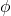or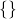• Finite and Infinite Set: A Set which is empty or consists of a definite number of elements is called finite set, otherwise the set is called infinite set.
• Singleton Set: A set consists of only one element.
• Subset: Let A and B be two sets. If every element of A is an element of B, then A is called a subset of B and we write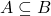. Here B is called superset of A and also written as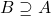• Every set is a subset of itself.
• The empty set is the subset of every set.
• Equal Sets: Two sets A and B are said to be equal if every element of A are also an element of B and every element of the set B are also in A. In other wordsand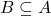implies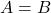.
• If A is a set with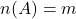, then the number of subsets of A are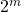.
• Power Set: The set of all subsets of a given set A is called the power set of A and is denoted by P(A).
• For example, if A = {1, 2, 3}, then P(A) = {, {1}, {2}, {3}, {1, 2} {1, 3}, {2, 3}, {1, 2, 3}}
• if A has n elements, then its power set P(A) contains exactly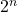elements
• Universal Set: A Set U that contains all sets in a given context is called the universal set.

#### Operations on Sets

• Union of Two Sets:   The union of two sets A and B, written as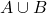(read as ‘A union B’), is the set consisting of all the elements which are either in A or in B or in both. i.e.,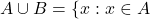or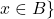• Intersection of Two Sets:   The intersection of two sets A and B, written as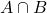(read as ‘A intersection B’), is the set consisting of all the elements which are both in A and  B. i.e.,and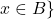• Difference of sets:   The difference of two sets A and B is the set of all the elements of A which do not belongs to B.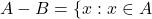and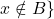• Symmetric difference of Two Sets:   The symmetric difference of two sets  A and B, is
••Complement of a Sets:   The complement of a set A, written as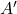or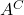, is the set consisting of all the elements which are not in A. i.e.,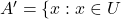and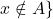#### Algebra of Set operation

• Idempotent Law: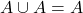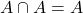• Identity Law:  For any set A,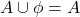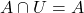• Commutative Law: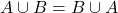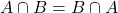• Associative  Law:   For any three sets A, B, C• Distributive  Law:   For any three sets A, B, C• De-morgan’s  Law:   For any two sets A and B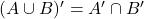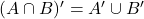• Cardinality or Order of a finite set:   The cardinality of a finite set is the number of elements in the set. The cardinality is denoted by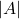.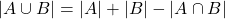•

For more understanding of the concept you can view some of the solved problems and MCQs regarding Set theory